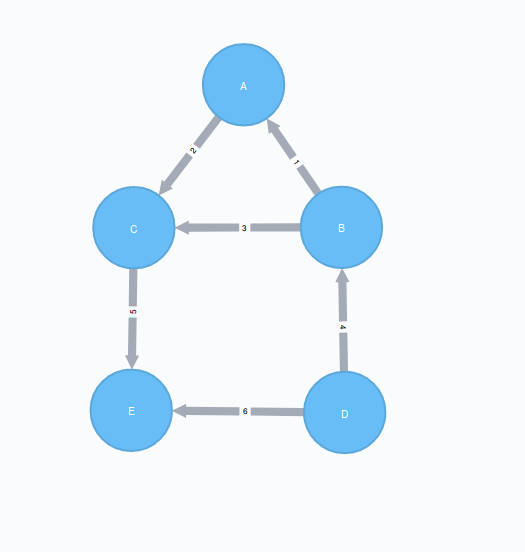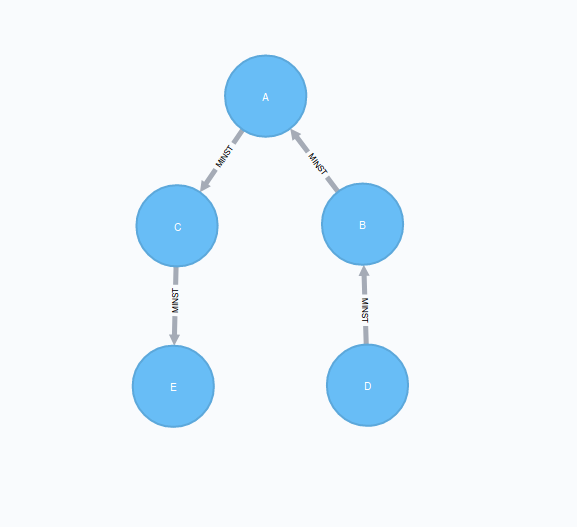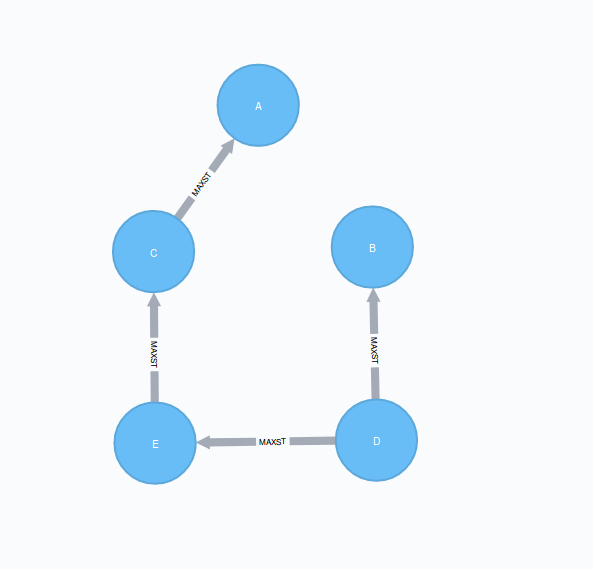# Minimum Weight Spanning Tree

The Minimum Weight Spanning Tree (MST) starts from a given node, and finds all its reachable nodes and the set of relationships that connect the nodes together with the minimum possible weight. Prim’s algorithm is one of the simplest and best-known minimum spanning tree algorithms. The K-Means variant of this algorithm can be used to detect clusters in the graph.

This algorithm is in the alpha tier. For more information on algorithm tiers, see Graph algorithms.

## 1. History and explanation

The first known algorithm for finding a minimum spanning tree was developed by the Czech scientist Otakar Borůvka in 1926, while trying to find an efficient electricity network for Moravia. Prim’s algorithm was invented by Jarnik in 1930 and rediscovered by Prim in 1957. It is similar to Dijkstra’s shortest path algorithm but, rather than minimizing the total length of a path ending at each relationship, it minimizes the length of each relationship individually. Unlike Dijkstra’s, Prim’s can tolerate negative-weight relationships.

The algorithm operates as follows:

• Start with a tree containing only one node (and no relationships).

• Select the minimal-weight relationship coming from that node, and add it to our tree.

• Repeatedly choose a minimal-weight relationship that joins any node in the tree to one that is not in the tree, adding the new relationship and node to our tree.

• When there are no more nodes to add, the tree we have built is a minimum spanning tree.

## 3. Constraints - when not to use the Minimum Weight Spanning Tree algorithm

The MST algorithm only gives meaningful results when run on a graph, where the relationships have different weights. If the graph has no weights, or all relationships have the same weight, then any spanning tree is a minimum spanning tree.

## 4. Syntax

The following will run the algorithm and write back results:
``````CALL gds.alpha.spanningTree.write(
graphName: string,
configuration: map
)
YIELD preProcessingMillis, computeMillis, writeMillis, effectiveNodeCount``````
The following will compute the minimum weight spanning tree and write the results:
``````CALL gds.alpha.spanningTree.minimum.write(
graphName: string,
configuration: map
)
YIELD preProcessingMillis, computeMillis, writeMillis, effectiveNodeCount``````
The following will compute the maximum weight spanning tree and write the results:
``````CALL gds.alpha.spanningTree.maximum.write(
graphName: string,
configuration: map
)
YIELD preProcessingMillis, computeMillis, writeMillis, effectiveNodeCount``````
Table 1. Configuration
Name Type Default Optional Description

startNodeId

Integer

null

no

The start node ID

relationshipWeightProperty

String

null

yes

Name of the relationship property to use as weights. If unspecified, the algorithm runs unweighted.

writeProperty

String

'mst'

yes

The relationship type written back as result

weightWriteProperty

String

n/a

no

The weight property of the `writeProperty` relationship type written back

Table 2. Results
Name Type Description

effectiveNodeCount

Integer

The number of visited nodes

preProcessingMillis

Integer

Milliseconds for preprocessing the data

computeMillis

Integer

Milliseconds for running the algorithm

writeMillis

Integer

Milliseconds for writing result data back

The following will run the k-spanning tree algorithms and write back results:
``````CALL gds.alpha.spanningTree.kmin.write(
graphName: string,
configuration: map
)
YIELD preProcessingMillis, computeMillis, writeMillis, effectiveNodeCount``````
``````CALL gds.alpha.spanningTree.kmax.write(
graphName: string,
configuration: map
)
YIELD preProcessingMillis, computeMillis, writeMillis, effectiveNodeCount``````
Table 3. Configuration
Name Type Default Optional Description

k

Integer

null

no

The result is a tree with `k` nodes and `k − 1` relationships

startNodeId

Integer

null

no

The start node ID

relationshipWeightProperty

String

null

yes

Name of the relationship property to use as weights. If unspecified, the algorithm runs unweighted.

writeProperty

String

'MST'

yes

The relationship type written back as result

weightWriteProperty

String

n/a

no

The weight property of the `writeProperty` relationship type written back

Table 4. Results
Name Type Description

effectiveNodeCount

Integer

The number of visited nodes

preProcessingMillis

Integer

Milliseconds for preprocessing the data

computeMillis

Integer

Milliseconds for running the algorithm

writeMillis

Integer

Milliseconds for writing result data back

## 5. Minimum Weight Spanning Tree algorithm sampleThe following will create a sample graph:
``````CREATE (a:Place {id: 'A'}),
(b:Place {id: 'B'}),
(c:Place {id: 'C'}),
(d:Place {id: 'D'}),
(e:Place {id: 'E'}),
(f:Place {id: 'F'}),
(g:Place {id: 'G'}),
The following will project and store a named graph:
``````CALL gds.graph.project(
'graph',
'Place',
{
properties: 'cost',
orientation: 'UNDIRECTED'
}
}
)``````

Minimum weight spanning tree visits all nodes that are in the same connected component as the starting node, and returns a spanning tree of all nodes in the component where the total weight of the relationships is minimized.

The following will run the Minimum Weight Spanning Tree algorithm and write back results:
``````MATCH (n:Place {id: 'D'})
CALL gds.alpha.spanningTree.minimum.write('graph', {
startNodeId: id(n),
relationshipWeightProperty: 'cost',
writeProperty: 'MINST',
weightWriteProperty: 'writeCost'
})
YIELD preProcessingMillis, computeMillis, writeMillis, effectiveNodeCount
RETURN preProcessingMillis, computeMillis, writeMillis, effectiveNodeCount;``````
To find all pairs of nodes included in our minimum spanning tree, run the following query:
``````MATCH path = (n:Place {id: 'D'})-[:MINST*]-()
WITH relationships(path) AS rels
UNWIND rels AS rel
WITH DISTINCT rel AS rel
RETURN startNode(rel).id AS source, endNode(rel).id AS destination, rel.writeCost AS cost``````Figure 1. Results
Table 5. Results
Source Destination Cost

D

B

4

B

A

1

A

C

2

C

E

5

The minimum spanning tree excludes the relationship with cost 6 from D to E, and the one with cost 3 from B to C. Nodes F and G aren’t included because they’re unreachable from D.

Maximum weighted tree spanning algorithm is similar to the minimum one, except that it returns a spanning tree of all nodes in the component where the total weight of the relationships is maximized.

The following will run the maximum weight spanning tree algorithm and write back results:
``````MATCH (n:Place{id: 'D'})
CALL gds.alpha.spanningTree.maximum.write('graph', {
startNodeId: id(n),
relationshipWeightProperty: 'cost',
writeProperty: 'MAXST',
weightWriteProperty: 'writeCost'
})
YIELD preProcessingMillis, computeMillis, writeMillis, effectiveNodeCount
RETURN preProcessingMillis,computeMillis, writeMillis, effectiveNodeCount;``````Figure 2. Results

### 5.1. K-Spanning tree

Sometimes we want to limit the size of our spanning tree result, as we are only interested in finding a smaller tree within our graph that does not span across all nodes. K-Spanning tree algorithm returns a tree with `k` nodes and `k − 1` relationships.

In our sample graph we have 5 nodes. When we ran MST above, we got a 5-minimum spanning tree returned, that covered all five nodes. By setting the `k=3`, we define that we want to get returned a 3-minimum spanning tree that covers 3 nodes and has 2 relationships.

The following will run the k-minimum spanning tree algorithm and write back results:
``````MATCH (n:Place{id: 'D'})
CALL gds.alpha.spanningTree.kmin.write('graph', {
k: 3,
startNodeId: id(n),
relationshipWeightProperty: 'cost',
writeProperty:'kminst'
})
YIELD preProcessingMillis, computeMillis, writeMillis, effectiveNodeCount
RETURN preProcessingMillis,computeMillis,writeMillis, effectiveNodeCount;``````
Find nodes that belong to our k-spanning tree result:
``````MATCH (n:Place)
WITH n.id AS Place, n.kminst AS Partition, count(*) AS count
WHERE count = 3
RETURN Place, Partition``````
Table 6. Results
Place Partition

A

1

B

1

C

1

D

3

E

4

Nodes A, B, and C are the result 3-minimum spanning tree of our graph.

The following will run the k-maximum spanning tree algorithm and write back results:
``````MATCH (n:Place{id: 'D'})
CALL gds.alpha.spanningTree.kmax.write('graph', {
k: 3,
startNodeId: id(n),
relationshipWeightProperty: 'cost',
writeProperty:'kmaxst'
})
YIELD preProcessingMillis, computeMillis, writeMillis, effectiveNodeCount
RETURN preProcessingMillis,computeMillis,writeMillis, effectiveNodeCount;``````
Find nodes that belong to our k-spanning tree result:
``````MATCH (n:Place)
WITH n.id AS Place, n.kmaxst AS Partition, count(*) AS count
WHERE count = 3
RETURN Place, Partition``````
Table 7. Results
Place Partition

A

0

B

1

C

3

D

3

E

3

Nodes C, D, and E are the result 3-maximum spanning tree of our graph.